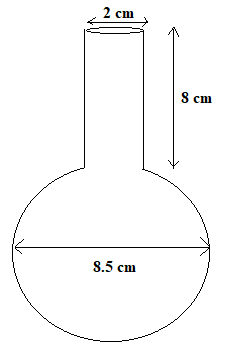QuestionAnswers

# A spherical glass vessel has a cylinder neck 8 cm long, 2 cm in diameter; the diameter of the spherical part is 8.5 cm. By measuring the amount of water it holds, a child finds its volume to be 345${\text{c}}{{\text{m}}^3}$. Check whether she is correct, taking the above as the inside measurements, and $\pi = 3.14$ .A. YesB. NoC. Less dataD. None of these.Hint: In this question, first make a diagram and write down the given data. Use the formula for volume of cylinder which is given by ${\text{V = }}\pi {{\text{r}}^2}h$ and then use the formula for finding volume of sphere which is given by ${\text{V = }}\dfrac{4}{3}\pi {{\text{r}}^3}$.

The diagram for the question is given below:In the question it is given:
Length of cylindrical part = 8 cm
Diameter of cylindrical part = 2 cm
$\therefore$ Radius of cylindrical part = $\dfrac{2}{2} = 1$cm
Diameter of spherical part = 8.5 cm
$\therefore$ Radius of spherical part = $\dfrac{{8.5}}{2} = 4.25$cm.

Now, we know that volume of cylinder is given by:
Volume of cylinder = $\pi {{\text{r}}^2}h{\text{ c}}{{\text{m}}^3}$ (1)
Putting the values in equation 1, we get:
Volume of cylindrical part = $\pi {{\text{r}}^2}h{\text{ = 3}}{\text{.14}} \times {{\text{1}}^3} \times {\text{8 = 25}}{\text{.12 c}}{{\text{m}}^3}$ .
Also, , we know that volume of cylinder is given by:
Volume of sphere = $\dfrac{4}{3}\pi {{\text{r}}^3}{\text{ c}}{{\text{m}}^3}$ (2)
Putting the values in equation 2, we get:
Volume of cylindrical part = $\dfrac{4}{3}\pi {{\text{r}}^3}{\text{ = }}\dfrac{4}{3} \times {\text{3}}{\text{.14}} \times {4.25^3}{\text{ = 321}}{\text{.39 c}}{{\text{m}}^3}$
Now, volume of water in vessel = volume of spherical part + volume of cylindrical part
= ${\text{321}}{\text{.39 c}}{{\text{m}}^3}$ +${\text{25}}{\text{.12 c}}{{\text{m}}^3}$ =${\text{346}}{\text{.51 c}}{{\text{m}}^3}$ .
So approximately the child is correct that the capacity of the vessel is ${\text{345 c}}{{\text{m}}^3}$ .
Therefore, option A is correct.

Note: In this type of question, you should know that the volume of liquid in a vessel is equal to the volume of the vessel. You should remember the formula for calculating the volume of cylinder and volume of sphere.
Area of Hollow CylinderVolume of a Triangular Prism FormulaArea of a Sector of a Circle FormulaArea of a Rhombus FormulaPerimeter of a Trapezoid FormulaSummary of a Photograph - PoemCentroid of a Trapezoid FormulaReconstitution of a Partnership FirmElectric Field of a SphereWhere There is a Will There is a Way EssayImportant Questions for CBSE Class 6 English A Pact with The Sun Chapter 10 - A Strange Wrestling MatchImportant Questions for CBSE Class 6 English A Pact with The Sun Chapter 8 - A Pact with the SunImportant Questions for CBSE Class 6 English Honeysuckle Chapter 8 - A Game of ChanceImportant Questions for CBSE Class 8 English Honeydew Chapter 8 - A Short Monsoon DiaryImportant Questions for CBSE Class 6 English A Pact with The Sun Chapter 1 - A Tale of Two BirdsCBSE Class 7 Science Water: A Precious Resource WorksheetsImportant Questions for CBSE Class 8 English Honeydew Chapter 7 - A Visit to CambridgeImportant Questions for CBSE Class 11 Business Studies Chapter 7 - Formation of a CompanyImportant Questions for CBSE Class 11 Physics Chapter 4 - Motion in a PlaneImportant Questions for CBSE Class 6 English Honeysuckle Chapter 5 - A Different Kind of SchoolCBSE Class 10 Hindi A Question Paper 2020Hindi A Class 10 CBSE Question Paper 2009Hindi A Class 10 CBSE Question Paper 2015Hindi A Class 10 CBSE Question Paper 2016Hindi A Class 10 CBSE Question Paper 2012Hindi A Class 10 CBSE Question Paper 2010Hindi A Class 10 CBSE Question Paper 2008Hindi A Class 10 CBSE Question Paper 2014Hindi A Class 10 CBSE Question Paper 2007Hindi A Class 10 CBSE Question Paper 2013RD Sharma Solutions for Class 9 Maths Chapter 19 - Surface Area and Volume of A Right Circular CylinderRS Aggarwal Class 8 Mathematics Solutions for Chapter-18 Area of a Trapezium and a PolygonNCERT Solutions for Class 9 English Moments Chapter 8 - A House is Not a HomeRS Aggarwal Solutions Class 8 Chapter-18 Area of a Trapezium and a Polygon (Ex 18B) Exercise 18.2RS Aggarwal Solutions Class 8 Chapter-18 Area of a Trapezium and a Polygon (Ex 18C) Exercise 18.3RS Aggarwal Solutions Class 8 Chapter-18 Area of a Trapezium and a Polygon (Ex 18A) Exercise 18.1NCERT Solutions for Class 12 Accountancy Chapter 3 - Reconstitution of a Partnership Firm: Admission of a PartnerNCERT Solutions for Class 12 English Kaliedoscope Poetry Chapter 4 Kubla Khan or a Vision in a Dream a FragmentNCERT Solutions for Class 12 Accountancy Chapter 4 - Reconstitution of a Partnership Firm:Retirement/Death of a PartnerNCERT Solutions for Class 6 English Honeysuckle Poem - A House, A Home